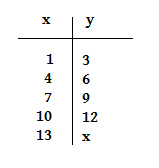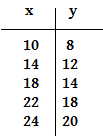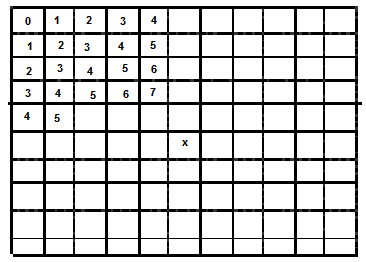Name:    3rd Grade Operations & Algebraic Thinking- Solve problems involving the four operations, and identify and explain patterns in arithmetic, Test 1

Multiple Choice
Identify the choice that best completes the statement or answers the question.

1.

What would be the next number in this pattern?   3    6    _____
 a. 3 c. 9 b. 12 d. 7

2.

What would be the next number in this pattern?   2    4    6   8   10  12     _____
 a. 14 c. 10 b. 20 d. 13

3.

What would be the next number in this pattern?   7    14   21        _____
 a. 22 c. 30 b. 28 d. 20

4.

What would be the next number in this pattern?   1   2   4   8      _____
 a. 12 c. 10 b. 6 d. 16

5.

What would be the next number in this pattern?  10  15  20   25    _____
 a. 20 c. 26 b. 50 d. 30

6.

What would be the next number in this pattern?  20  _____   40   50
 a. 30 c. 25 b. 50 d. 21

7.

What number is missing in this pattern?    3   6  9   ____  15   18
 a. 12 c. 14 b. 10 d. 3

8.

What number is missing in this pattern?    20   16    _____  8  4  0
 a. 4 c. 14 b. 12 d. 10

9.

What number is missing in this pattern?   77    79    81  _____
 a. 82 c. 83 b. 85 d. 75

10.What number will go in spot x?
 a. 13 c. 14 b. 15 d. 9

11.What is the pattern in this graph?
 a. + 4 c. - 2 b. + 1 d. + 2

12.

What number is missing in this pattern?   6    12    18   24    18    12   _____
 a. 0 c. 18 b. 6 d. 9

13.What number would go in square X?
 a. 11 c. 9 b. 8 d. 10